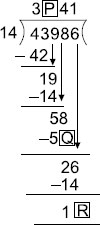# User Forum

Subject :IMO    Class : Class 4

Find P + Q - R.A 7
B 5
C 4
D 3

WHY B?

Class : Class 7

## Ans 2:

Class : Class 5

Class : Class 1

(B):Here, P = 1, Q = 6, R = 2
So, P + Q – R = 1 + 6 – 2 = 5

Class : Class 7

## Ans 5:

Class : Class 5
(B):Here, P=1, Q=6,R=2So, P Q-R=16-2=5

## Ans 6:

Class : Class 4
this question is wrong the correct answer is A

## Ans 7:

Class : Class 7
answer ha b not a you must have took the 8 in the position of 6 like I did by mistake check again please retry and concentratethats it

Class : Class 6
B

## Ans 9:

Class : Class 2
Yes the answer is B Because P=1,Q=6 so 1 6=7

## Ans 10:

Class : Class 5
(B):Here,P=1,Q=6,R=2So,P Q-R=1 6-2=5

Class : Class 6